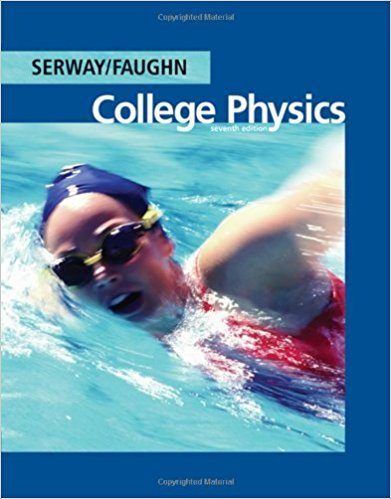×
Get Full Access to College Physics - 7 Edition - Chapter 18 - Problem 18.9
Get Full Access to College Physics - 7 Edition - Chapter 18 - Problem 18.9

×

# Consider the circuit shown in Figure P18.9. Find (a) theISBN: 9780495113690 154

## Solution for problem 18.9 Chapter 18

College Physics | 7th Edition

• Textbook Solutions
• 2901 Step-by-step solutions solved by professors and subject experts
• Get 24/7 help from StudySoup virtual teaching assistantsCollege Physics | 7th Edition

4 5 1 259 Reviews
23
3
Problem 18.9

Consider the circuit shown in Figure P18.9. Find (a) the current in the 20.0-resistor and (b) the potential difference between points a and b.

Step-by-Step Solution:
Step 1 of 3

Step 2 of 3

Step 3 of 3

##### ISBN: 9780495113690

This full solution covers the following key subjects: Circuit, consider, current, difference, figure. This expansive textbook survival guide covers 30 chapters, and 2135 solutions. Since the solution to 18.9 from 18 chapter was answered, more than 242 students have viewed the full step-by-step answer. This textbook survival guide was created for the textbook: College Physics, edition: 7. The full step-by-step solution to problem: 18.9 from chapter: 18 was answered by , our top Physics solution expert on 11/15/17, 04:28PM. College Physics was written by and is associated to the ISBN: 9780495113690. The answer to “Consider the circuit shown in Figure P18.9. Find (a) the current in the 20.0-resistor and (b) the potential difference between points a and b.” is broken down into a number of easy to follow steps, and 24 words.

Unlock Textbook Solution